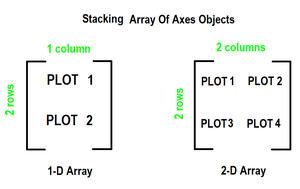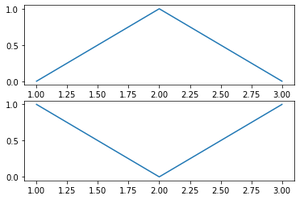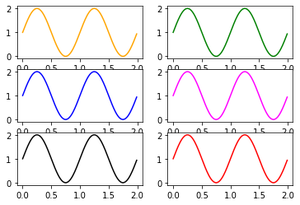GFG App
Open AppBrowser
Continue

# How to create multiple subplots in Matplotlib in Python?

To create multiple plots use matplotlib.pyplot.subplots method which returns the figure along with Axes object or array of Axes object. nrows, ncols attributes of subplots() method determine the number of rows and columns of the subplot grid.

By default, it returns a figure with a single plot. For each axes object i.e plot we can set title (set via set_title()), an x-label (set via set_xlabel()), and a y-label set via set_ylabel()).

Let’s see how this works

1. When we call the subplots() method by stacking only in one direction it returns a 1D array of axes object i.e subplots.
2. We can access these axes objects using indices just like we access elements of the array. To create specific subplots, call matplotlib.pyplot.plot() on the corresponding index of the axes. Refer to the following figure for a better understandingExample 1: 1-D array of subplots

## Python3

 `# importing library` `import` `matplotlib.pyplot as plt`   `# Some data to display` `x ``=` `[``1``, ``2``, ``3``]` `y ``=` `[``0``, ``1``, ``0``]` `z ``=` `[``1``, ``0``, ``1``]`   `# Creating 2 subplots` `fig, ax ``=` `plt.subplots(``2``)`   `# Accessing each axes object to plot the data through returned array` `ax[``0``].plot(x, y)` `ax[``1``].plot(x, z)`

Output :subplots_fig1

Example2: Stacking in two directions returns a 2D array of axes objects.

## Python3

 `# importing library` `import` `matplotlib.pyplot as plt` `import` `numpy as np`   `# Data for plotting` `x ``=` `np.arange(``0.0``, ``2.0``, ``0.01``)` `y ``=` `1` `+` `np.sin(``2` `*` `np.pi ``*` `x)`   `# Creating 6 subplots and unpacking the output array immediately` `fig, ((ax1, ax2), (ax3, ax4), (ax5, ax6)) ``=` `plt.subplots(``3``, ``2``)` `ax1.plot(x, y, color``=``"orange"``)` `ax2.plot(x, y, color``=``"green"``)` `ax3.plot(x, y, color``=``"blue"``)` `ax4.plot(x, y, color``=``"magenta"``)` `ax5.plot(x, y, color``=``"black"``)` `ax6.plot(x, y, color``=``"red"``)`

Output :Subplots_fig2

My Personal Notes arrow_drop_up